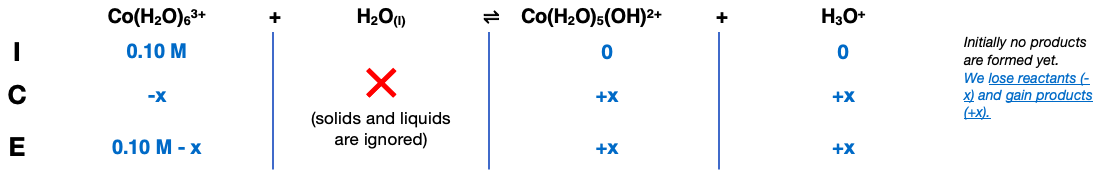# Problem: Calculate the pH of a 0.10-M CoCl 3 solution. The Ka value for Co(H2O)63+ is 1.0 X 10 -5.

###### FREE Expert Solution

We are being asked to determine the pH of 0.10 M CoCl3

CoCl3 dissociates in water in the following manner

• CoCl3 -> Co3+ + 3 Cl-
• Cl is neutral

Co is hydrated and forms Co(H2O)63+ which releases H+

Co(H2O)63+ + H2O -> Co(H2O)5(OH)2+ + H3O+ Ka = 1.0 X 10 -5

From this, we can construct an ICE table. Remember that liquids are ignored in the ICE table.The Ka expression for Co(H2O)63+ is:

Liquids are ignored. Note that each concentration is raised by the stoichiometric coefficient: [Co(H2O)63+], [H3O+] and [Co(H2O)5(OH)2+] are raised to 1.

79% (409 ratings)###### Problem Details

Calculate the pH of a 0.10-M CoCl 3 solution. The Ka value for Co(H2O)63+ is 1.0 X 10 -5.

Frequently Asked Questions

What scientific concept do you need to know in order to solve this problem?

Our tutors have indicated that to solve this problem you will need to apply the Weak Acids concept. If you need more Weak Acids practice, you can also practice Weak Acids practice problems.

What professor is this problem relevant for?

Based on our data, we think this problem is relevant for Professor Ande's class at FAU.

What textbook is this problem found in?

Our data indicates that this problem or a close variation was asked in Chemistry: An Atoms First Approach - Zumdahl Atoms 1st 2nd Edition. You can also practice Chemistry: An Atoms First Approach - Zumdahl Atoms 1st 2nd Edition practice problems.